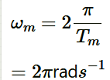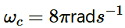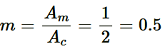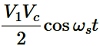# Chapter-15 Communications System

Question1. Which of the following frequencies will be suitable for beyond-the-horizon communication using sky waves?

(a) 10 kHz

(b) 10 MHz

(c) 1 GHz

(d) 1000 GHz

Solution :

(b) 10 MHz

For beyond-the-horizon communication, it is necessary for the signal waves to travel a large distance. 10 KHz signals cannot be radiated efficiently because of the antenna size. The high energy signal waves (1GHz − 1000 GHz) penetrate the ionosphere. 10 MHz frequencies get reflected easily from the ionosphere. Hence, signal waves of such frequencies are suitable for beyond-the-horizon communication.

Question2. Frequencies in the UHF range normally propagate by means of:

(a) Ground waves.

(b) Sky waves.

(c) Surface waves.

(d) Space waves.

Solution :
(d) Space waves

Owing to its high frequency, an ultra high frequency (UHF) wave can neither travel along the trajectory of the ground nor can it get reflected by the ionosphere. The signals having UHF are propagated through line-of-sight communication, which is nothing but space wave propagation.

Question3. Digital signals

(i) Do not provide a continuous set of values,

(ii) Represent values as discrete steps,

(iii) Can utilize binary system, and

(iv) Can utilize decimal as well as binary systems.

Which of the above statements are true?

(a) (i) and (ii) only

(b) (ii) and (iii) only

(c) (i), (ii) and (iii) but not (iv)

(d) All of (i), (ii), (iii) and (iv).

Solution :
(c)  A digital signal uses the binary (0 and 1) system for transferring message signals. Such a system cannot utilise the decimal system (which corresponds to analogue signals). Digital signals represent discontinuous values.

Question4. Is it necessary for a transmitting antenna to be at the same height as that of the receiving antenna for line-of-sight communication? A TV transmitting antenna is 81m tall. How much service area can it cover if the receiving antenna is at the ground level?

Solution :
Line-of-sight communication means that there is no physical obstruction between the transmitter and the receiver. In such communications it is not necessary for the transmitting and receiving antennas to be at the same height.

Height of the given antenna, h = 81 m

Radius of earth, R = 6.4 × 106 m

For range, d = (2Rh)½, the service area of the antenna is given by the relation:

A = πd

= π (2Rh)

= 3.14 × 2 × 6.4 × 106 × 81

= 3255.55 × 106 m2

= 3255.55

∼ 3256 km2

Question5. A carrier wave of peak voltage 12 V is used to transmit a message signal. What should be the peak voltage of the modulating signal in order to have a modulation index of 75%?

Solution :
Amplitude of the carrier wave, Ac = 12 V

Modulation index, m = 75% = 0.75

Amplitude of the modulating wave = Am

Using the relation for modulation index:

Question6. A modulating signal is a square wave, as shown in Fig. 15.14.

The carrier wave is given by:

(i) Sketch the amplitude modulated waveform

(ii) What is the modulation index?

Solution :
It can be observed from the given modulating signal that the amplitude of the modulating signal, Am = 1 V

It is given that the carrier wave c (t) = 2 sin (8πt)

∴Amplitude of the carrier wave, Ac = 2 V

Time period of the modulating signal Tm = 1 s

The angular frequency of the modulating signal is calculated as:……(i)

The angular frequency of the carrier signal is calculated as:…….(ii)

From equations (i) and (ii), we get:

The amplitude modulated waveform of the modulating signal is shown in the following figure.

(ii)Modulation index,Question7.For an amplitude modulated wave, the maximum amplitude is found to be 10 V while the minimum amplitude is found to be 2 V. Determine the modulation index μ. What would be the value of μ if the minimum amplitude is zero volt?

Solution :
Maximum amplitude, Amax = 10 V

Minimum amplitude, Amin = 2 V

Modulation index μ, is given by the relation:

Question8. Due to economic reasons, only the upper sideband of an AM wave is transmitted, but at the receiving station, there is a facility for generating the carrier. Show that if a device is available which can multiply two signals, then it is possible to recover the modulating signal at the receiver station.

Solution :
Let ωc and ωs be the respective frequencies of the carrier and signal waves.

Signal received at the receiving station, V = V1 cos (ωc + ωs)t

Instantaneous voltage of the carrier wave, Vin = Vc cos ωct

At the receiving station, the low-pass filter allows only high frequency signals to pass through it. It obstructs the low frequency signal ωs. Thus, at the receiving station, one can record the modulating signal, which is the signal frequency.

Get 30% off your first purchase!

X
error: Content is protected !!
Scroll to Top